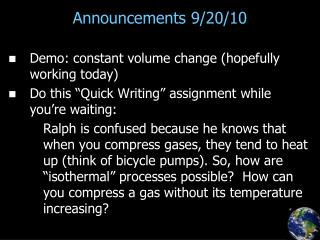DownloadDownload PresentationAnnouncements 9/20/10

# Announcements 9/20/10

Télécharger la présentation## Announcements 9/20/10

- - - - - - - - - - - - - - - - - - - - - - - - - - - E N D - - - - - - - - - - - - - - - - - - - - - - - - - - -
##### Presentation Transcript

1. Announcements 9/20/10 • Demo: constant volume change (hopefully working today) • Do this “Quick Writing” assignment while you’re waiting: Ralph is confused because he knows that when you compress gases, they tend to heat up (think of bicycle pumps). So, how are “isothermal” processes possible? How can you compress a gas without its temperature increasing?

2. Thought question (ungraded) • How will the temperature of the gas change during this process from A to B? • Increase • Decrease • First increase, then decrease • First decrease, then increase • Stay the same

3. Reading quiz (graded) • What is “CV”? • heat capacity • mass-pacity • molar heat capacity • molar heat capacity, but only for constant volume changes • a detailed resumé

4. Thought question (ungraded) • Which should be larger, the molar heat capacity for constant volume changes or the molar heat capacity for constant pressure changes? (Hint: Think of the First Law.) • constant volume • constant pressure • they are the same • it depends on the temperature

5. CV and CP • Constant volume change (monatomic): W = 0 Eint = Qadded (3/2)nRT = Qadded Compare to definition of C: Qadded = nCVDT (like Qadded = mcT) CV = (3/2)R • Constant pressure change • What’s different? • result: (monatomic) CP = (5/2)R • What would be different for gases with more degrees of freedom?

6. Reading quiz (graded) • What does gamma equal in the equation for an adiabatic process: • CP + CV • CP - CV • CV - CP • CV / CP • CP / CV

8. Thought question • How much do you think the temperature of the air in this room would change by if I compressed it adiabatically by a factor of 10? (Vf = V0/10) • less than 0.1 degree C • about 0.1 degrees C • about 1 degree C • about 10 degrees C • more than 10 degrees C

9. Demo/Video • Video: adiabatic cotton burner • Demo: freeze spray • Video: adiabatic expansion

10. Derivation of PVg (for Monatomic) Eint = Qadded + Won (3/2) nRT = -integral(PdV) (3/2) nRdT = -PdV (3/2) nR d(PV/nR) = -PdV (3/2) (PdV + VdP) = -PdV (3/2) VdP = -(5/2) PdV dP/P = -(5/3) dV/V lnP = (-5/3)lnV + constant lnP = ln(V-5/3) + constant P = constant  V-5/3 (it’s a different constant) P V5/3 = constant What’s different if diatomic?

11. Thought question • Which of the curves on the PV diagram below is most likely to represent an isothermal compression, followed by an adiabatic expansion back to the initial volume?

12. Thought questions • What would be the molar specific heat for an adiabatic process? (Hint: think of Q = nCDT.) • CV • CV + R • CV + 2R • CV - R • none of the above • What would be the molar specific heat for an isothermal process? (Same hint.) • CV • CV + R • CV + 2R • CV - R • none of the above

13. Water/steam “saturation curve”: ideal gas?

14. Water/steam “saturation curve”: ideal gas?

15. Water/steam “saturation curve”: ideal gas?

16. Water/steam “saturation curve”: ideal gas?